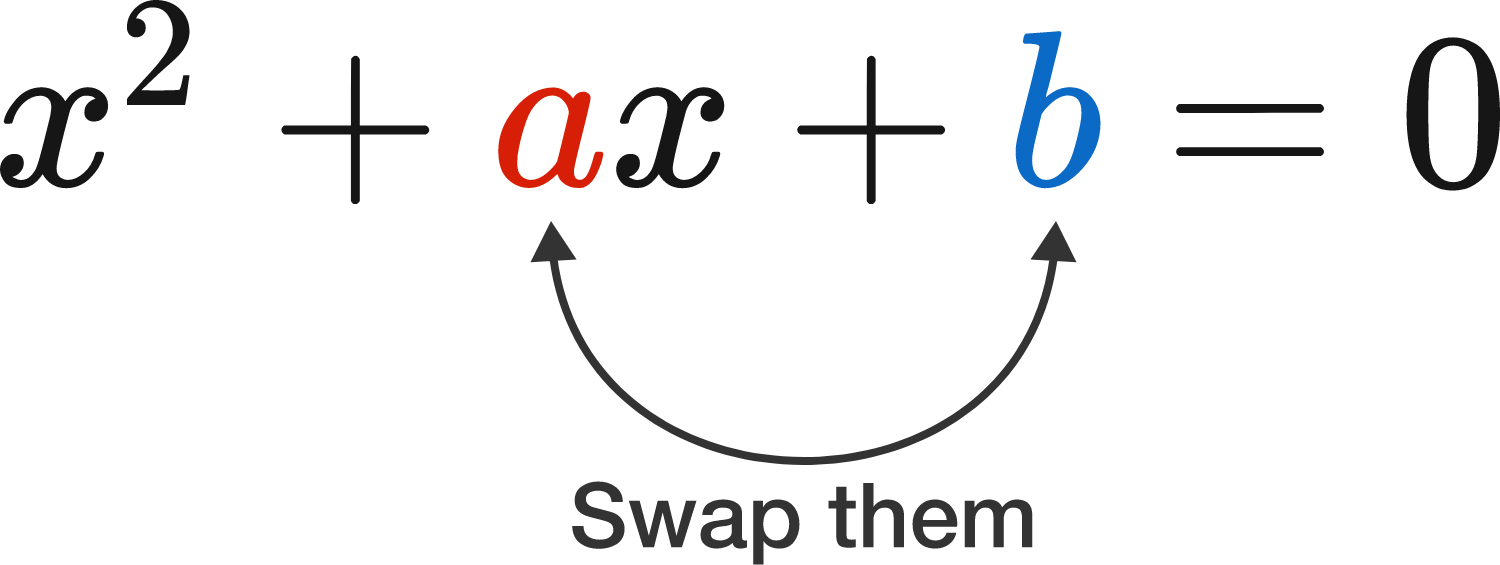# Swapping Symbols With Minimal Difference

Algebra Level 3A student notices that the roots of the equation $x^2+bx+a=0$ are each one less than the roots of the equation $x^2+ax+b=0$.

Find the value of $a+b$.

×